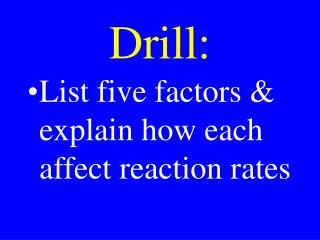# Drill: - PowerPoint PPT PresentationDownload PresentationDrill:

Drill:Download Presentation## Drill:

- - - - - - - - - - - - - - - - - - - - - - - - - - - E N D - - - - - - - - - - - - - - - - - - - - - - - - - - -
##### Presentation Transcript

1. Drill: • List five factors & explain how each affect reaction rates

2. Review Drill&Check HW

3. CHM II HW • Review PP-19 & 20 • Complete the attached worksheet & turn it in tomorrow • Lab Thursday

4. Chemical Equilibria

5. Equilibrium • The point at which the rate of a forward reaction = the rate of its reverse reaction

6. Equilibrium • The concentration of all reactants & products become constant at equilibrium

7. Equilibrium • Because concentrations become constant, equilibrium is sometimes called steady state

8. Equilibrium • Reactions do not stop at equilibrium, forward & reverse reaction rates become equal

9. Reaction • aA(aq)+ bB(aq) pP(aq)+ qQ(aq) • Ratef = kf[A]a[B]b • Rater = kr[P]p[Q]q • At equilibrium, Ratef = Rater • kf[A]a[B]b = kr[P]p[Q]q

10. At equilibrium, Ratef = Rater kf[A]a[B]b = kr[P]p[Q]q kf /kr = ([P]p[Q]q)/ ( [A]a[B]b) kf /kr = Kc = Keq in terms of concentration Kc = ([P]p[Q]q)/ ( [A]a[B]b)

11. All Aqueous aA + bB pP+ qQ

12. Equilibrium Expression ( Products)p (Reactants)r Keq=

13. Drill: Solve Rate Expr: X + Y M + N fast 3M + N 2G fast 2N K fast 2G + K Prod. slow

14. Drill: Solve Rate Expr: X + Y M + N fast 3M + N 2G fast 2N K fast 2G + K Prod. slow

15. Work from the slow step up

16. Drill: Solve Rate Expr: X + Y M + N fast 3M + N 2G fast 2N K fast 2G + K Prod. slow

17. 1) Cancel K & G

18. Drill: Solve Rate Expr: X + Y M + N fast 3M + N 2G fast 2N K fast 2G + K Prod. slow

19. Cancel K & GTriple rxn 1 & cancel

20. Drill: Solve Rate Expr: 3X + 3Y 3M + 3N 3M + N 2G fast 2N K fast 2G + K Prod. slow

21. Solve Rate Law A + B C + D fast 4 C + A 2G fast 2 K 4D + B fast G + K 2 Q + 2 W fast Q + W Prod. slow

22. Drill: Solve Rate Law A + B C + D fast 4 C + A 2G fast 2 K 4D + B fast G + K 2 Q + 2 W fast Q + W Prod. slow

23. 1) Reverse step 3

24. Drill: Solve Rate Law A + B C + D fast 4 C + A 2G fast 4 D + B 2K fast G + K 2 Q + 2 W fast Q + W Prod. slow

25. Reverse Rxn 3Divide Rxn 4 by 2 & cancel

26. Drill: Solve Rate Law A + B C + D fast 4 C + A 2G fast 4 D + B 2K fast G/2 + K/2 Q + W fast Q + W Prod. slow

27. Drill: Solve Rate Law A + B C + D fast C + A/4 G/2 fast D + B/4 K/2 fast G/2 + K/2 Q + W fast Q + W Prod. slow

28. Reverse Rxn 3Divide Rxn 4 by 2 & cancelDivide Rxns 2 & 3 by 4 & cancelAdd the rxns

29. 5/4 A + 5/4 B  ProductRate = k[A]5/4[B]5/4

30. Review & Collect Drill & HW

31. CHM II HW • Review PP-19 & 20 • Remember M. S.

32. Equilibrium Applications • When K >1, [P] > [R] • When K <1, [P] < [R]

33. Equilibrium Calculations • Kp = Kc(RT)Dngas

34. Equilibrium Expression • Reactants or products not in the same phase are not included in the equilibrium expression

35. Equilibrium Expression aA(s)+ bB(aq) cC(aq)+ dD(aq) [C]c [D]d [B]b Keq=

36. Reaction Mechanism • When one of the intermediates anywhere in a reaction mechanism is altered, all intermediates are affected

37. Reaction Mechanism • 1) A + B <---> C + D • 2) C + D <---> E + K • 3) E + K <---> H + M • 4) H + M <----> P

38. Lab Results • % 100 80 60 40 • RT 5.21 8.42 11.9 21.7 • WR 2.75 4.23 7.96 11.2

39. aA(aq) + bB(aq) pP(aq) + qQ(aq) ReactionQuotient where [A], [B], [P], and [Q] are molarities at any time. Q = K only at equilibrium.

40. Drill: NH3 H2 + N2 At a certain temperature at equilibrium Pammonia = 4.0 Atm, Phydrogen = 2.0 Atm, & Pnitrogen = 5.0 Atm. Calculate Keq:

41. Review & Collect Drill & HW

42. CHM II HW • Review PP 19 & 20 • Complete the attached assignment & turn it in tomorrow

43. aA(aq) + bB(aq) pP(aq) + qQ(aq) ReactionQuotient where [A], [B], [P], and [Q] are molarities at any time. Q = K only at equilibrium.

44. Equilibrium Applications • When K > Q, the reaction goes forward • When K < Q, the reaction goes in reverse

45. Equilibrium Calculations • aA + bB  pP + qQ • Stoichiometry is used to calculate the theoretical yield in a one directional rxn

46. Equilibrium Calculations • aA + bB  pP + qQ • In equilibrium rxns, no reactant gets used up; so, calculations are different

47. Equilibrium Calculations • Set & balance rxn • Assign amounts with x • Write eq expression • Substitute amounts • Solve for x